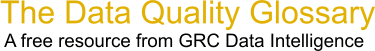Choose Index below for a list of all words and phrases defined in this glossary.

# Probability

index | Index

## Probability - definitions

Probability - Probability refers to the chance of something happening, or the fraction of occurrences over a large number of trials. Probability can range from 0 (no chance) to 1 (full certainty).

[Category=Data Quality ]

These advertisers support this free service

probability - [statistics] A measure of the likelihood that a particular outcome, such as a spatial pattern or event, will occur given a set of possible outcomes. Probability values range from 0 for impossible outcomes to 1 for completely certain outcomes. The probability that a tossed coin will land heads-up, for example, is 0.5, since landing heads-up is one of two possible outcomes.

[Category=Geospatial ]

Source: esri, 18 July 2012 11:44:42, http://support.esri.com/en/knowledgebase/GISDictionary/term/abbreviationData Quality Glossary.  A free resource from GRC Data Intelligence. For comments, questions or feedback: dqglossary@grcdi.nl